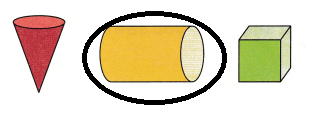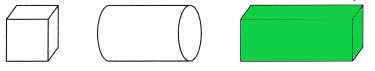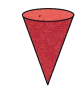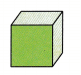# McGraw Hill Math Grade 1 Chapter 11 Lesson 9 Answer Key Solid Figures

All the solutions provided in McGraw Hill Math Grade 1 Answer Key PDF Chapter 11 Lesson 9 Solid Figures as per the latest syllabus guidelines.

## McGraw-Hill Math Grade 1 Answer Key Chapter 11 Lesson 9 Solid Figures

Identify

Question 1.
Circle the cylinder.Cylinder.
Explanation:
A cylinder is round and has a top and bottom in the shape of a circle.
so, circled the cylinder.

Question 2.
Color the rectangular prism green.Explanation:
It is also called a cuboid. A rectangular prism has six faces, and all the faces are in a rectangle shape and have twelve edges.
so, colored the rectangular prism.

Circle the name of the solid figure. Then write the name.

Question 3.cube
cone
cylinder
Cone
Explanation:
A cone is a 3D shape consisting of a circular base and once continuous curved surface tapering to a point

Question 4.cylinder
cube
cone